SURFACES of the EXTENDED EUCLIDEAN SPACE

I. Definition and intrinsic properties of surfaces

Definition: Surface (surface patch) c is any connected non-empty subset of the extended Euclidean space,
c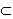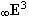, which is a continuous mapping of the connected region WR2.
Analytic representation of the surface is a point function

p(u,v)=(x(u,v), y(u,v), z(u,v), h(u,v))

defined, continuous and at least once differentiable on W.

Value of the point function for (a,b)ÎW is the point on the surface determined by
the quadruple of homogeneous
coordinates

P(a,b)=p(a,b)=(x(a,b), y(a,b), z(a,b), h(a,b))

Numbers (a,b)ÎW are called parametric - curvilineare coordinates of the surface point.
For real points on the surface h(a,b)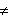0.
If the region W is a regular region, we speak about surface patch.
In the computer processing, the parametrisation of the surface is usually chosen on
the region W=<0,1>2
and we speak about the surface patch over the unit square.
Point function defines the orientation of the surface patch
c.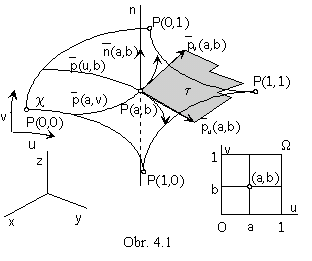Points on the surface patch,
with the curvilinear coordinates equal to 0 or 1,
are denoted as corner points of the surface patch

P(0,0), P(1,0), P(0,1), P(1,1).

For constant values of one variable u=a, or v=b, we receive from the point function of two variables the point function of one variable determining the curve located on the surface, which is called parametric v-curve, or u-curve. Parametric curves form two systems of curves located on the surface. Each curve from one system intersects all curves from the other system. Two parametric curves from different systems have a common point located on the surface, curvilinear coordinates of this point are coresponding constant values of parameters u and v

p(a,v)Çp(u,b)=p(a,b)=P(a,b)

Parametric curve segments located on the surface patch with constant values of variables u and v equal to 0 or 1,
are called boundary curve segments of the surface patch.
Surface patch boundary curve segments from different parametric systems intersect in the patch corner points, Fig. 4. 1.

Partial derivative of the point function p(u,v) is the vector function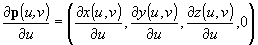, or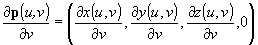determining for u=a, or v=b the tangent vector to the parametric u-curve, or v-curve in the point P(a,b),
denoted as
pu(a,b), or pv(a,b).

Point on the surface, in which any from the partial derivatives pu(a,b), or pv(a,b) of the surface point function is the zero vector, or these two vectors are linearly dependent, is called the singular point.

In the regular point P(a,b), there are tangent vectors to the parametric curves non-zero and linearly independent,
they define a unique tangent plane
t to the surface in the regular point.

Tangent plane t in the regular point P(a,b) to the surface containes tangent lines to all curves located on the surface and passing through the tangent point.

Vector

n(a,b)=pu(a,b)´pv(a,b)

Is called normal vector to the surface in the regular point P(a,b).
It is perpendicular to the tangent plane and in the case of the positive orientation of the surface it is oriented to the opposite halfspace from the tangent plane than the surface.
Line determined by the normal vector and passing through the point P(a,b) is called normal to the surface. Normal to the surface is perpendicular to tangent lines to all curves located on the surface and passing through the point P(a,b), which are located in the tangent plane
t.

Point on the surface is called elliptic point , if the tangent plane to the surface in this point does not contain any other point of the surface in the neighbourhood of the tangent point. This neigbourhood on the surface is located in one halspace determined by the tangent plane (Fig. 4. 2 a). All points on the sphere or on the ellipsoid are elliptic points.

Point on the surface is called parabolic point , if the tangent plane to the surface in this point contains a curve located on the surface, it is tangent to the surface in this curve (or it ntersects the surface in the curve, while the tangent point is the cuspidal point on the tangent curve). Neighbourhood of the tangent curve on the surface is located in one halfspace determined by the tangent plane (Fig. 4. 2 b). All points on the cylindrical or the conical surface are parabolic points.

Point on the surface is called hyperbolic point , if the tangent plane to the surface in this point intersects the surface in the intersection curve, while the tagent point is the double point on the intersection curve. Surface is located in both halfspaces determined by the tangent plane (Fig. 4. 2 c). All points on the hyperboloid are hyperbolic points.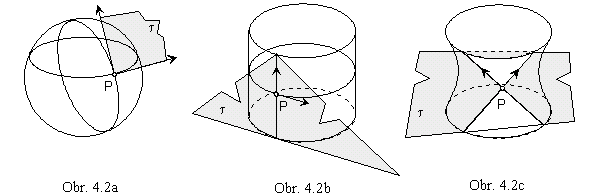Torus is the surface, on which all types of points are located (Fig. 4. 3).
It can be created by revolving a basic circle k(S, r ) about the axis, which is located in the plane of the basic circle.
All points on circles l and l´, created by revolutions of the points P and P´ from the basic circle k about the axis of revolution o (and located in the same distance from the axis o as the centre S of the basic circle k), are parabolic points.
Tangent planes
t and t´ are perpendicular to the axis o and they are tangent to the torus in the circles l and l´.
All points on the torus, created by revolution of the circular arc PP´ (clockwise oriented) are elliptic points, tangent planes to the torus in these points have no more common points with the torus, but the tangent point.
All points on the torus, created by revolution of the circular arc PP´ (anti-clockwise oriented) are hyperbolic points, tangent planes to the torus in these points intersect torus in the curve with the double point in the tangent point.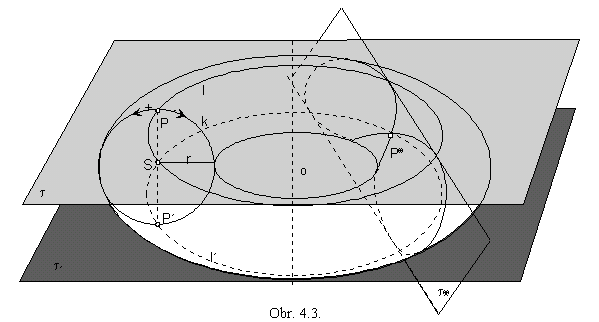Vector

puv(a,b) =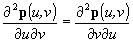of the mixed second partial derivative of the surface point function
is called
twist vector to the surface in the regular point P(a,b).
It represents the "curvature" of the surface in the neighbourhood of the given point.
Point, in which the twist vector is a zero vector, puv(a,b) = 0
, is the point of inflection,
surface is in the neighbourhood of this point a part of the plane.

II. Problems on surfaces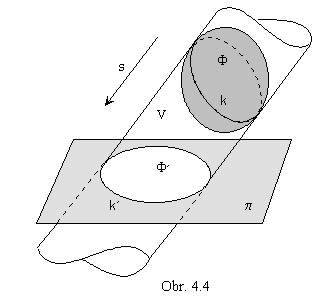1. Views of surfaces

View of the surface is the set of views of all points on the surface. In the parallel projection, all projecting lines of points on the surface create a cylindrical space. View of the surface is the intersection of this space with the image plane. Tangent cylindrical surface subscribed to the surface F, with lines parallel to the direction of the projection, is called the projecting cylindrical surface V.
Tangent curve k of the surface
F and projecting cylindrical surface V is the contoure curve of the surface F.
Image of the contoure curve k of the surface
F in the image plane p is the curve k´ - outline of the surface F, outline of the view F´ in the image plane (obr. 4.4).

2. Point on the surface - tangent plane to the surface - normal to the surface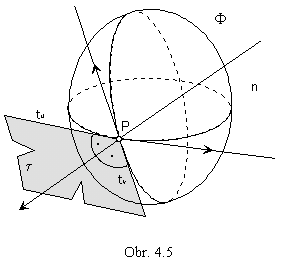If the point is located on the surface, then it is located on some curve on the surface.
There is located one parametric
u-curve and one parametric v-curve of the surface in any surface point.
Tangent plane t in the regular point P on the surface F
is determined uniquelly by tangent lines tu and tv
to the parametric curves located on the surface
in the common point P (Fig. 4. 5).
Normal n to the surface is the line perpendicular
to the tangent plane
t in the point P on the surface F,
it is perpendicular to both tangent lines to the surface parametric curves located in the surface point P
n
^ tu         n^ tv         n^ t.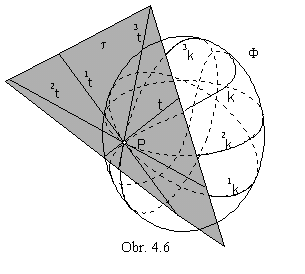3. Curve on the surface - tangent line in the given point

An infinite number of different curves can be located
on the surface in any given surface point.
Tangent lines to all these curves on the surface
F
in the point P
ÎF are located in the tangent plane t
to the surface in the given point P
.
If the curve k on the surface
F is passing through the point P,
the tangent line t to this curve in the point P
is the line of the tangent plane
t (Fig. 4. 6).
P Îik,   ikF,
it is the tangent line to the curve ik in the point P,     itt

4. Planar intersection of the surface - tangent line to the intersection in the given point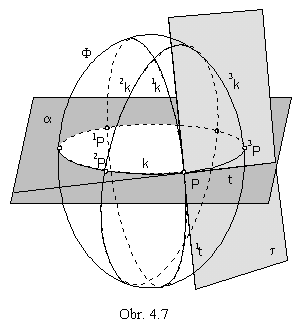Intersection curve k is the set of all intersection points of curves in one system of surface parametric curves with the intersection plane.
If all curves 1k, 2k, . .. , ik are from the same system of parametric curves on the surface
F,
points 1k
Ç a = 1P, 2kÇ a = 2P, ..., ikÇ a = iP
are points on the intersection curve k of the surface
F
and the plane
a (obr. 4.7).
Tangent line t to the intersection curve k in the point P
is the line located in the intersection plane
a
(intersection curve k is the plane curve,
osculating plane
a containes all tangent lines to the curve k).
Curve k is located on the surface,
therefore the tangent line t to the curve in the point P
is located in the tangent plane
t to the surface in the tangent point P.
ta,      tt ,      then      t=a Ç t

5. Intersection points of a line and surface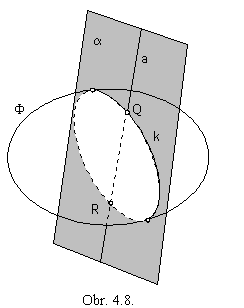Line can be located into an arbitrary plane, which intersects the surface.
Intersection curve and the given line (as geometric figures located in the same plane) can have an empty intersection - in this case the line does not intersect the surface, or they have common points, which are intersection points of the line and the surface (Fig. 4. 8).
Algorithm can be symbolically denoted in the following three steps
:

1. aa

2. aÇF=k

3. kÇa={ } ,     then aÇF={ }, line does not intersect the surface
or

kÇa={Q, R, ...} ,     then a ÇF={ Q, R, ...}

6. Intersection of surfaces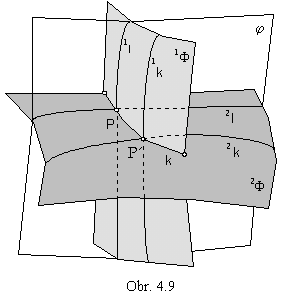Intersection curve of two surfaces
is the set of intersection points of pairs of curves,
each of them located on a different surface.

1l1F,    2l2F,    1l Ç 2l =P,

P
Îk,    k=12F

Curves 1l, 2l having common points
must be located on one surface
j (Fig. 4. 9).
Auxhiliary surface
j
intersects the surface
1F in the curve 1l
and the surface 2
F in the curve 2l,

j Ç1F=1l, j Ç2F=2l.

For the easy determination of the intersection curves
1l, 2l , it is convinient to choose elementary auxhiliary surfaces, for instance planes, or spheres.
Choosing the suitable system of auxhiliary surfaces intersecting given
surfaces 1F, 2F
in systems of curves
1li, 2li the points Pi on the intersection curve k are constructed
as all common of curves 1li, 2li
.

7. Regular mappings of surfaces

Regular mapping of the surface 1F to the surface 2F is calles development (isometric mapping), if it preserves invariant the length of curve segments on surfaces (Fig. 4. 10).

12F,       1A1B1k ® 2A2B2k,      |1A1B|=|2A2B|

Surface is developable, if it can be developed to the plane.

Regular mapping of one surface to another one is called similarity (conform mapping), if it preserves the size of angles which form curve segments on surfaces (angle of two curve segments is equal to the angle of tangent lines to the curves in their common point) (Fig. 4. 11).

1F ® 2F      1k ® 2k      1l ® 2l            |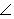1k 1l| = |1a 1b| = |2k 2l| = |2a 2b|

For any two surfaces 1F, 2F there exists such neighbourhood 1O of the point 1PÎ1F, for which there can be found
a neighbourhood
2O of its image 2PÎ2F, that the mapping of these neighbourhoods 1O on 2O is a conform mapping.

Some types of maps are conform mappings of the part of the Earth to the plane.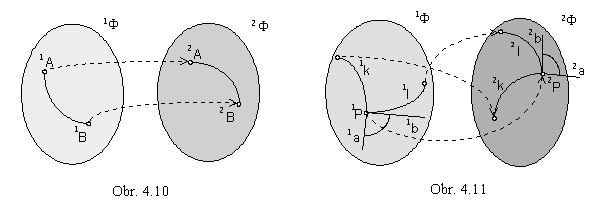III. Modelling of surfaces

Synthetic representations of surfaces in the Creative space can be in three different types.

1. (a curve segment, a class of geometric transformations)

Curve segment is analytically represented
by a point function of the given properties on the interval <0,1>
(see Lecture 2.).

Analytic representation of the class of geometric transformations
is the matrix - function T
(v) of type 4´4, defined on the interval <0,1>,
with elements of the given properties (see Lecture 2.).

Modelled surface patch is analytically represented
by a point function defined on the region
W=<0,1>2
and satisfying the required properties

p(u, v)=r(u).T(v)

2. (a surface patch, a geometric transformation)

From the given surface patch analytically represented
by the point function q(
u, v)
of the required properties on the region
W there can be created a surface patch.

Applied geometric transformation is defined
by the regular square matrix
T of rank four with real elements.

Modelled surface patch can be congruent to the basic one (in metric transformations),
or it can be an affine, or a projective image of the basic figure.
Point function of the required properties
defined on the region
W and representing the surface analytically is in the from

p(u, v)=q(u, v) . T

3. (an ordered set of points or curve segments, an approximation or an interpolation)

Map M of the modelled surface patch - analytic representation of the basic figure
is the matrix of type n
´m,
its elements are analytic representations of the elements of the basic figure,
quadruples of the homogeneous coordinates of points,
or point functions of curve segments.

Approximation or interpolation are analytically represented
by matrices I(u) ans I(v) of types 1
´n and 1´m,
with elements in the form of the polynomial functions Pli(u) of degree n-1
and Pli(v) of degree m-1 satisfying the given conditions.

Interpolation surface patch is analytically represented
by a point function

p(u,v)= I(u) . M . I . T(v),

defined and satisfying the required properties on the region W=<0,1>2.